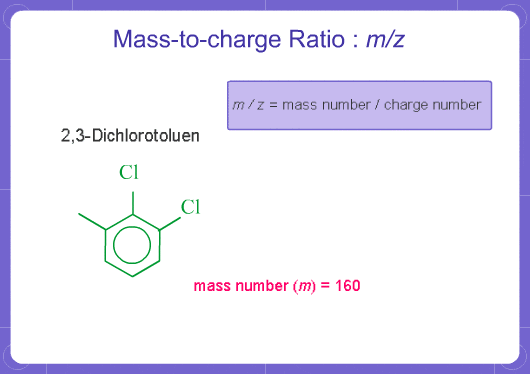# What does m/z mean?

## 1. BASIC MASS SPECTROMETRY

### 01. What does m/z mean?

M stands for mass and Z stands for charge number of ions. In mass analysis, an electron is taken from molecules to create single charged ions. If two electrons are removed, double charged ions are produced. The number of electrons removed is the charge number (for positive ions). m/z represents mass divided by charge number and the horizontal axis in a mass spectrum is expressed in units of m/z. Since z is almost always 1 with GCMS, the m/z value is often considered to be the mass.Back to Index